# Complement of an Event

Experiment 1: A spinner has 4 equal sectors colored yellow, blue, green and red. What is the probability of landing on a sector that is not red after spinning this spinner?Sample Space: {yellow, blue, green, red}

Probability: The probability of each outcome in this experiment is one fourth. The probability of landing on a sector that is not red is the same as the probability of landing on all the other colors except red.

 P(not red) = 1 + 1 + 1 = 3 4 4 4 4

In Experiment 1, landing on a sector that is not red is the complement of landing on a sector that is red.

Definition: The complement of an event A is the set of all outcomes in the sample space that are not included in the outcomes of event A. The complement of event A is represented by(read as A bar).

Rule: Given the probability of an event, the probability of its complement can be found by subtracting the given probability from 1.

 P() = 1 - P(A)

You may be wondering how this rule came about. In the last lesson, we learned that the sum of the probabilities of the distinct outcomes within a sample space is 1. For example, the probability of each of the 4 outcomes in the sample space above is one fourth, yielding a sum of 1. Thus, the probability that an outcome does not occur is exactly 1 minus the probability that it does. Let's look at Experiment 1 again, using this subtraction principle.Experiment 1: A spinner has 4 equal sectors colored yellow, blue, green and red. What is the probability of landing on a sector that is not red after spinning this spinner?

Sample Space: {yellow, blue, green, red}

Probability:

 P(not red) = 1 - P(red)
 = 1 - 1 4
 = 3 4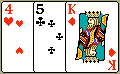Experiment 2: A single card is chosen at random from a standard deck of 52 playing cards. What is the probability of choosing a card that is not a king?

Probability:

 P(not king) = 1 - P(king)
 = 1 - 4 52
 = 48 52
 = 12 13

Experiment 3: A single 6-sided die is rolled. What is the probability of rolling a number that is not 4?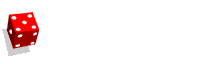Probability:

 P(not 4) = 1 - P(4)
 = 1 - 1 6
 = 5 6Experiment 4: A single card is chosen at random from a standard deck of 52 playing cards. What is the probability of choosing a card that is not a club?

Probability:

 P(not club) = 1 - P(club)
 = 1 - 13 52
 = 39 52
 = 3 4Experiment 5: A glass jar contains 20 red marbles. If a marble is chosen at random from the jar, what is the probability that it is not red?

Probability:

 P(not red) = 1 - P(red)
 = 1 - 1
 = 0

Note: This is an impossible event.

Summary: The probability of an event is the measure of the chance that the event will occur as a result of the experiment. The probability of an event A, symbolized by P(A), is a number between 0 and 1, inclusive, that measures the likelihood of an event in the following way:

• If P(A) > P(B) then event A is more likely to occur than event B.
• If P(A) = P(B) then events A and B are equally likely to occur.
• If event A is impossible, then P(A) = 0.
• If event A is certain, then P(A) = 1.
• The complement of event A is.    P() = 1 - P(A)

### Exercises

Directions: Read each question below. Select your answer by clicking on its button. Feedback to your answer is provided in the RESULTS BOX. If you make a mistake, choose a different button.

 1. A glass jar contains 5 red, 3 blue and 2 green jelly beans. If a jelly bean is chosen at random from the jar, what is the probability that it is not blue?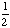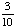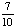None of the above. RESULTS BOX:
 2. A student is chosen at random from a class of 16 girls and 14 boys. What is the probability that the student chosen is not a girl?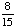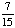1 None of the above. RESULTS BOX:
 3. A number from 1 to 5 is chosen at random. What is the probability that the number chosen is not odd?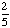0 None of the above. RESULTS BOX:
 4. If a number is chosen at random from the following list, what is the probability that it is not prime? 2, 3, 5, 7, 11, 13, 17, 19 1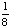0 None of the above. RESULTS BOX:
 5. If a single 6-sided die is rolled, what is the probability of rolling a number that is not 8?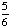1 0 None of the above. RESULTS BOX: